## Example Questions

### Example Question #1 : How To Subtract Rational Expressions With Different Denominators

Choose the answer which best simplifies the following expression: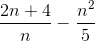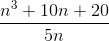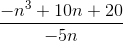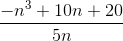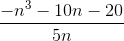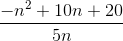Explanation:

To simplify this problem, multiply each term by the denominator of the other over itself: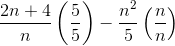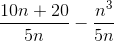Now that both terms share a denominator, you can subtract:### Example Question #1 : How To Subtract Rational Expressions With Different Denominators

Choose the answer which best simplifies the following expression: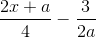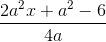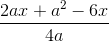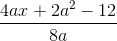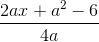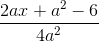Explanation:

To solve this problem, first multiply each term of the original expression by the denomenator of the other over itself: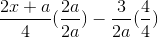Then you will have two terms with a common denomenator: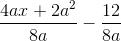### Example Question #11 : Rational Expressions

Choose the answer which best simplifies the expression below: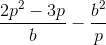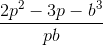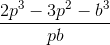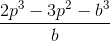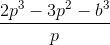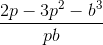Explanation:

To simplify this problem, multiply each term by the denomenator of the other over itself: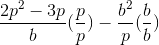Then you will yield terms with a like denomenator, which can be combined: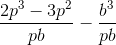### Example Question #291 : Algebra

Choose the answer which best simplifies the expression below: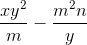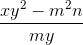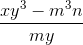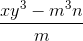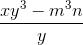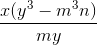Explanation:

To simplify the expression, first multiply each term by the denomenator of the other over itself: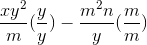Then you yield terms with common denomenators, which can be combined: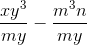### Example Question #1 : How To Subtract Rational Expressions With Different Denominators

Choose the answer which best simplifies the following expression: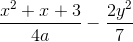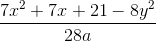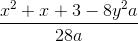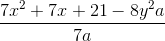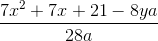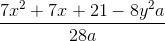Explanation:

To simplify, first multiply each of the terms by the denomenator of the other over itself: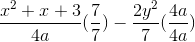Then you will get terms with a common denomenator, which can be combined: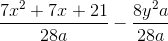### Example Question #11 : Rational Expressions

Choose the answer which best simplifies the expression below: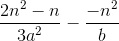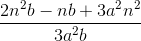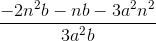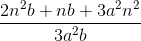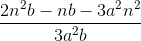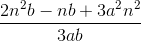Explanation:

To simplify, first multiply each of the terms by the denomenator of the other over itself: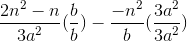You will yield terms with a common denomenator, which can be combined: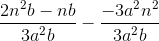### Example Question #2 : How To Subtract Rational Expressions With Different Denominators

Choose the answer which best simplifies the expression below: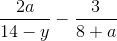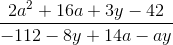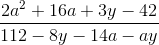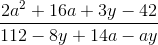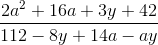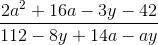Explanation:

To simplify, multiply each of the terms by the denomenator of the other, over itself: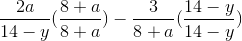You will yield terms with a common denomenator, which can be combined: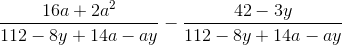### Example Question #1 : Rational Expressions

If √(ab) = 8, and a= b, what is a?

10

4

2

16

64

4

Explanation:

If we plug in a2 for b in the radical expression, we get √(a3) = 8. This can be rewritten as a3/2 = 8. Thus, loga 8 = 3/2. Plugging in the answer choices gives 4 as the correct answer.

### Example Question #1 : How To Evaluate Rational Expressions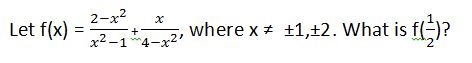–37/15

–9/5

37/15

–11/5

9/5

–11/5

Explanation: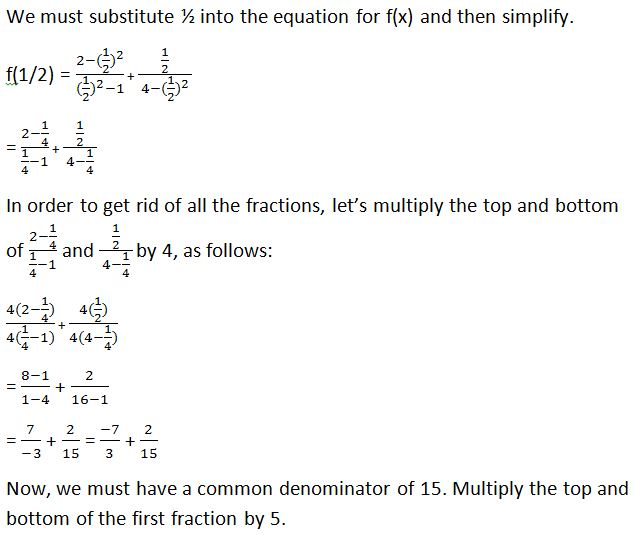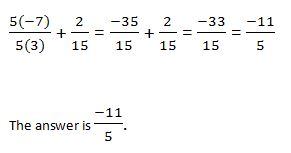### Example Question #11 : Expressions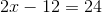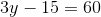Find the product ofand.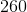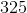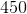Explanation:

Solve the first equation for.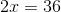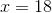Solve the second equation for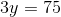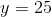The final step is to multiplyand.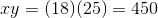### All GRE Math Resources